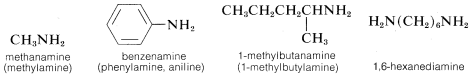# 7.8: Amines

$$\newcommand{\vecs}{\overset { \rightharpoonup} {\mathbf{#1}} }$$ $$\newcommand{\vecd}{\overset{-\!-\!\rightharpoonup}{\vphantom{a}\smash {#1}}}$$$$\newcommand{\id}{\mathrm{id}}$$ $$\newcommand{\Span}{\mathrm{span}}$$ $$\newcommand{\kernel}{\mathrm{null}\,}$$ $$\newcommand{\range}{\mathrm{range}\,}$$ $$\newcommand{\RealPart}{\mathrm{Re}}$$ $$\newcommand{\ImaginaryPart}{\mathrm{Im}}$$ $$\newcommand{\Argument}{\mathrm{Arg}}$$ $$\newcommand{\norm}{\| #1 \|}$$ $$\newcommand{\inner}{\langle #1, #2 \rangle}$$ $$\newcommand{\Span}{\mathrm{span}}$$ $$\newcommand{\id}{\mathrm{id}}$$ $$\newcommand{\Span}{\mathrm{span}}$$ $$\newcommand{\kernel}{\mathrm{null}\,}$$ $$\newcommand{\range}{\mathrm{range}\,}$$ $$\newcommand{\RealPart}{\mathrm{Re}}$$ $$\newcommand{\ImaginaryPart}{\mathrm{Im}}$$ $$\newcommand{\Argument}{\mathrm{Arg}}$$ $$\newcommand{\norm}{\| #1 \|}$$ $$\newcommand{\inner}{\langle #1, #2 \rangle}$$ $$\newcommand{\Span}{\mathrm{span}}$$$$\newcommand{\AA}{\unicode[.8,0]{x212B}}$$

The word "amine" is derived from ammonia, and the class of compounds known as amines therefore are commonly named as substituted ammonias. In this system, primary amines ($$RNH_2$$), having only one substituent on nitrogen, are named with the substituent as a prefix. More systematic nomenclature appends -amine to the longest chain, as for alcohols:Secondary ($$R_2NH$$) and tertiary amines ($$R_3N$$), which have two and three substituents on nitrogen, commonly are named as N-substituted amines. As for substituted amides, N is included to indicate that the substituent is on the nitrogen atom unless there is no ambiguity as to where the substituent is located. Systematic nomenclature of secondary and tertiary amines is related to the systematic ether nomenclature discussed in Section 7-3:As a substituent, the $$-NH_2$$ group is called amino. N-Substituted amino groups are named accordingly:$$^1$$Alphabetical order puts chloro- ahead of chloroethyl-.